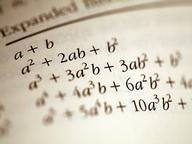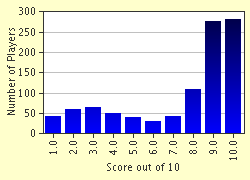FREE! Click here to Join FunTrivia. Thousands of games, quizzes, and lots more!# Linear Equations in One Variable Quiz

### A quiz on linear equations in one variable to re-live old memories of high school math. All answers are positive integers. You might want to make a note of the answers as you progress. Enjoy!

A multiple-choice quiz by achernar. Estimated time: 3 mins.

Author
achernar
Time
3 mins
Type
Multiple Choice
Quiz #
118,222
Updated
Feb 24 23
# Qns
10
Difficulty
Average
Avg Score
7 / 10
Plays
14948
Awards
Top 20% Quiz
Last 3 plays: Guest 171 (3/10), Guest 165 (4/10), Guest 174 (0/10).
1. If a + 54 = 5a - 18, then the value of 'a' is... Hint

12
18
24
27

#### NEXT>

2. If [(2b)/3] - 17 = b - 34, then the value of 'b' is... Hint

23
51
19
41

#### NEXT>

3. If 12c - 2(c + 1) = 8c + 22, then the value of 'c' is... Hint

16
12
24
30

#### NEXT>

4. If 3d - 5 = [(4d)/3] + 40, then the value of 'd' is... Hint

27
36
9
54

#### NEXT>

5. If (7e + 5)/e = 26/3, then the value of 'e' is... Hint

3
9
6
12

#### NEXT>

6. If (9 + 3f)/(4f + 22) = 1/2, then the value of 'f' is... Hint

2
51
42
17

#### NEXT>

7. If (1 - 3g)/5 = -(g - 5), then the value of 'g' is... Hint

6
12
7
14

#### NEXT>

8. If 9h - 4 = (16h + 4)/2, then the value of 'h' is... Hint

5
2
6
4

#### NEXT>

9. If (4i - 8)/5 = i - 4, then the value of 'i' is... Hint

12
256
3
64

#### NEXT>

10. Now, using the values for 'a', 'b', 'c', 'd', 'e', 'f', 'g', 'h' and 'i' which you have obtained from the equations in questions 1 - 9, find the value of 'x' in the following equation:

[(b/e + x)/x][(fc - g)/i] = (d/x + a + e)/h

Most Recent Scores
Sep 21 2023 : Guest 171: 3/10
Sep 14 2023 : Guest 165: 4/10
Sep 11 2023 : Guest 174: 0/10
Sep 09 2023 : Guest 99: 9/10
Sep 08 2023 : Guest 223: 6/10
Sep 03 2023 : Dagny1: 8/10
Sep 02 2023 : Guest 175: 4/10
Sep 01 2023 : Edzell_Blue: 7/10
Aug 30 2023 : Guest 157: 0/10

Score DistributionQuiz Answer Key and Fun Facts
1. If a + 54 = 5a - 18, then the value of 'a' is...

a + 54 = 5a - 18
=> 54 + 18 = 5a - a
=> 72 = 4a
=> a = 72/4
=> a = 18
2. If [(2b)/3] - 17 = b - 34, then the value of 'b' is...

[(2b)/3] - 17 = b - 34
=> (2b)/3 - b = -34 + 17
=> (2b - 3b)/3 = -17
=> -b/3 = -17
=> -b = -17 * 3
=> b = 51
3. If 12c - 2(c + 1) = 8c + 22, then the value of 'c' is...

12c - 2(c + 1) = 8c + 22
=> 12c - 2c - 2 = 8c + 22
=> 12c - 2c - 8c = 22 + 2
=> 2c = 24
=> c = 24/2
=> c = 12
4. If 3d - 5 = [(4d)/3] + 40, then the value of 'd' is...

3d - 5 = [(4d)/3] + 40
=> 3d - (4d)/3 = 40 + 5
=> (9d - 4d)/3 = 45
=> (5d)/3 = 45
=> 5d = 45 * 3
=> 5d = 135
=> d = 135/5
=> d = 27
5. If (7e + 5)/e = 26/3, then the value of 'e' is...

(7e + 5)/e = 26/3
=> 21e + 15 = 26e
=> 26e - 21e = 15
=> 5e = 15
=> e = 15/5
=> e = 3
6. If (9 + 3f)/(4f + 22) = 1/2, then the value of 'f' is...

(9 + 3f)/(4f + 22) = 1/2
=> 18 + 6f = 4f + 22
=> 6f - 4f = 22 - 18
=> 2f = 4
=> f = 4/2
=> f = 2
7. If (1 - 3g)/5 = -(g - 5), then the value of 'g' is...

(1 - 3g)/5 = -(g - 5)
=> (1 - 3g)/5 = -g + 5
=> 1 - 3g = -5g + 25
=> -3g + 5g = 25 - 1
=> 2g = 24
=> g = 24/2
=> g = 12
8. If 9h - 4 = (16h + 4)/2, then the value of 'h' is...

9h - 4 = (16h + 4)/2
=> 18h - 8 = 16h + 4
=> 18h - 16h = 4 + 8
=> 2h = 12
=> h = 12/2
=> h = 6
9. If (4i - 8)/5 = i - 4, then the value of 'i' is...

(4i - 8)/5 = i - 4
=> 4i - 8 = 5i - 20
=> 5i - 4i = 20 - 8
=> i = 12
10. Now, using the values for 'a', 'b', 'c', 'd', 'e', 'f', 'g', 'h' and 'i' which you have obtained from the equations in questions 1 - 9, find the value of 'x' in the following equation: [(b/e + x)/x][(fc - g)/i] = (d/x + a + e)/h

From questions 1 to 9, the values for the all variables 'a' to 'i' that you get are: 'a'=18, 'b'=51, 'c'=12, 'd'=27, 'e'=3, 'f'=2, 'g'=12, 'h'=6, and 'i'=12. Now, by putting these values in the equation in the question, you get:
[(51/3 + x)/x][(2 * 12 - 12)/12] = (27/x + 18 + 3)/6
=> [(17 + x)/x][(24 - 12)/12] = (27/x + 21)/6
=> [(17 + x)/x][12/12] = (27/x + 21)/6
=> [(17 + x)/x] * 1 = (27/x + 21)/6
=> (17 + x)/x = (27/x + 21)/6
=> 102 + 6x = 27 + 21x
=> 21x - 6x = 102 - 27
=> 15x = 75
=> x = 75/15
=> x = 5
Phew! I hope you enjoyed solving these equations (regardless of how well you did)!
Source: Author achernar

This quiz was reviewed by FunTrivia editor CellarDoor before going online.
Any errors found in FunTrivia content are routinely corrected through our feedback system.
Related Quizzes